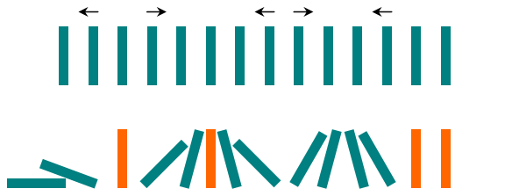0838. Push Dominoes

# 838. Push Dominoes#

## 题目 #

There are N dominoes in a line, and we place each domino vertically upright.

In the beginning, we simultaneously push some of the dominoes either to the left or to the right.After each second, each domino that is falling to the left pushes the adjacent domino on the left.

Similarly, the dominoes falling to the right push their adjacent dominoes standing on the right.

When a vertical domino has dominoes falling on it from both sides, it stays still due to the balance of the forces.

For the purposes of this question, we will consider that a falling domino expends no additional force to a falling or already fallen domino.

Given a string “S” representing the initial state. S[i] = ‘L’, if the i-th domino has been pushed to the left; S[i] = ‘R’, if the i-th domino has been pushed to the right; S[i] = ‘.’, if the i-th domino has not been pushed.

Return a string representing the final state.

Example 1:

``````
Input: ".L.R...LR..L.."
Output: "LL.RR.LLRRLL.."

``````

Example 2:

``````
Input: "RR.L"
Output: "RR.L"
Explanation: The first domino expends no additional force on the second domino.

``````

Note:

• 0 <= N <= 10^5
• String dominoes contains only ‘L’, ‘R’ and ‘.’

## 代码 #

``````
package leetcode

// 解法一
func pushDominoes(dominoes string) string {
d := []byte(dominoes)
for i := 0; i < len(d); {
j := i + 1
for j < len(d)-1 && d[j] == '.' {
j++
}
push(d[i : j+1])
i = j
}
return string(d)
}

func push(d []byte) {
first, last := 0, len(d)-1
switch d[first] {
case '.', 'L':
if d[last] == 'L' {
for ; first < last; first++ {
d[first] = 'L'
}
}
case 'R':
if d[last] == '.' || d[last] == 'R' {
for ; first <= last; first++ {
d[first] = 'R'
}
} else if d[last] == 'L' {
for first < last {
d[first] = 'R'
d[last] = 'L'
first++
last--
}
}
}
}

// 解法二
func pushDominoes1(dominoes string) string {
dominoes = "L" + dominoes + "R"
res := ""
for i, j := 0, 1; j < len(dominoes); j++ {
if dominoes[j] == '.' {
continue
}
if i > 0 {
res += string(dominoes[i])
}
middle := j - i - 1
if dominoes[i] == dominoes[j] {
for k := 0; k < middle; k++ {
res += string(dominoes[i])
}
} else if dominoes[i] == 'L' && dominoes[j] == 'R' {
for k := 0; k < middle; k++ {
res += string('.')
}
} else {
for k := 0; k < middle/2; k++ {
res += string('R')
}
for k := 0; k < middle%2; k++ {
res += string('.')
}
for k := 0; k < middle/2; k++ {
res += string('L')
}
}
i = j
}
return res
}

``````